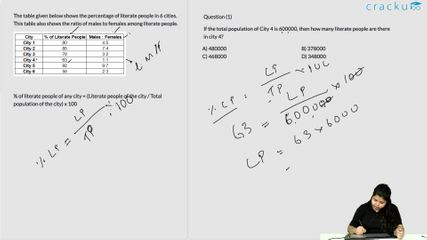### 2017 SSC CGL 09 Aug Shift-2 Question 99

Instructions

The table given below shows the percentage of literate people in 6 cities. This table also shows the ratio of males to females among literate people.

Question 99

# If there are 259210 literate females in City 5, then what is the total population of City 5?

Solution

Let total literate population in city 5 = $$16x$$

=> Number of literate females in city 5 = $$\frac{7}{16}\times16x=7x$$

According to ques, => $$7x=259210$$

=> $$x=\frac{259210}{7}=37030$$

% of literate population in city 5 = 92%

=> Total population in city 5 = $$16x\times\frac{100}{92}$$

= $$\frac{1600}{92}\times37030=644,000$$

=> Ans - (A)

### View Video Solution